# Scientific Notation Definition and Explanation of Scientific notation

• Slides: 18Scientific NotationDefinition and Explanation of Scientific notation • A positive number is written in scientific notation if it is written in the form: a. b…. . x 10 r where 1 < a < 10 and r is a power of 10. a. b…. . is the coefficient 10 is the base r is the exponent Examples: 4. 5 x 103 6. 7112 x 10 -2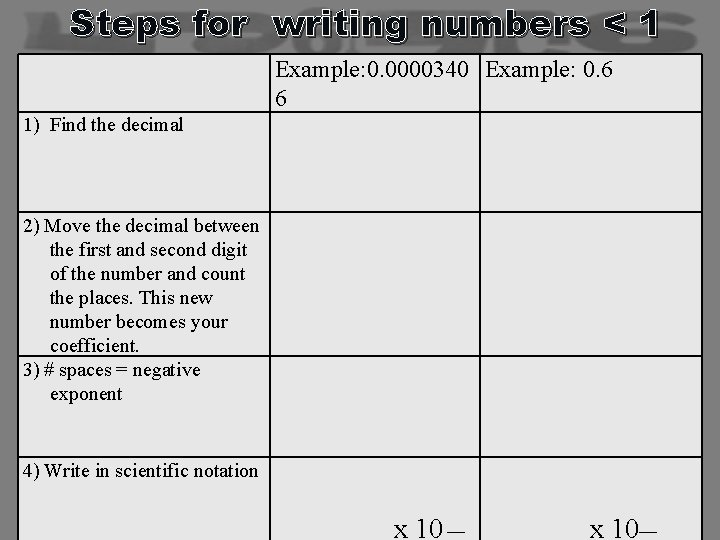Steps for writing numbers < 1 Example: 0. 0000340 Example: 0. 6 6 1) Find the decimal 2) Move the decimal between the first and second digit of the number and count the places. This new number becomes your coefficient. 3) # spaces = negative exponent 4) Write in scientific notation ____ x 10 __ _______ x 10__Examples 1) 0. 00000073 = 2) 0. 000903 = 3) 0. 00631 = 4). 555900 = 5) 0. 8 = 6) 0. 030 =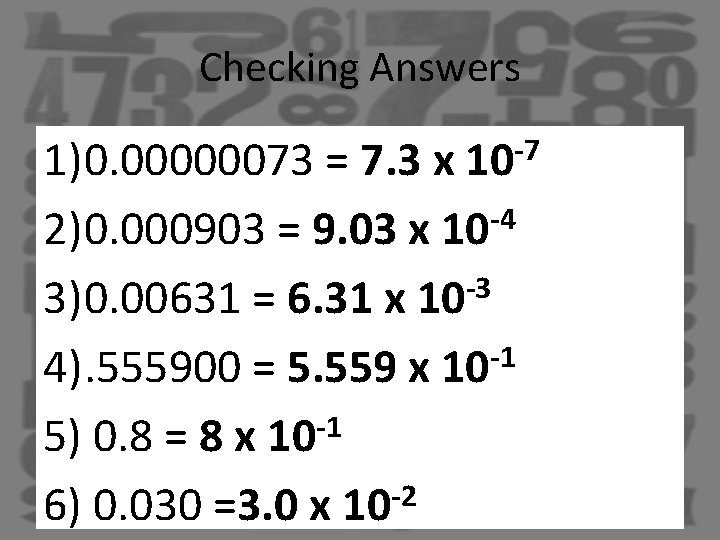Checking Answers 1)0. 00000073 = 7. 3 x 10 -7 2)0. 000903 = 9. 03 x 10 -4 -3 3)0. 00631 = 6. 31 x 10 -1 4). 555900 = 5. 559 x 10 5) 0. 8 = 8 x 10 -1 -2 6) 0. 030 =3. 0 x 10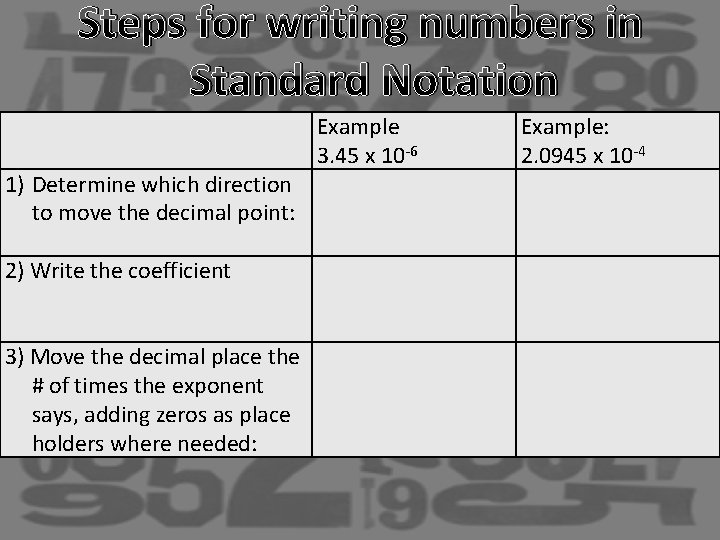Steps for writing numbers in Standard Notation 1) Determine which direction to move the decimal point: 2) Write the coefficient 3) Move the decimal place the # of times the exponent says, adding zeros as place holders where needed: Example 3. 45 x 10 -6 Example: 2. 0945 x 10 -4Examples 1) 2) 3) 4) 5) 6) 7) 1. 2142 x 10 -4 = 9. 5149 x 10 -6 3. 526 X 10 -2= 7. 2030 X 100 = 9. 50000 X 10 -8 = 8. 001 X 10 -4= 3. 8 X 10 -7=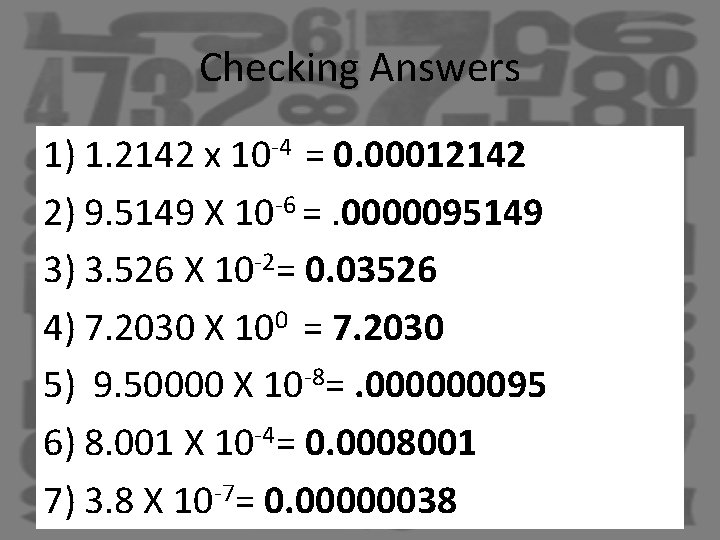Checking Answers 1) 1. 2142 x 10 -4 = 0. 00012142 2) 9. 5149 X 10 -6 =. 0000095149 3) 3. 526 X 10 -2= 0. 03526 4) 7. 2030 X 100 = 7. 2030 5) 9. 50000 X 10 -8=. 000000095 6) 8. 001 X 10 -4= 0. 0008001 7) 3. 8 X 10 -7= 0. 00000038SummaryExit TicketCalculations with Scientific Notation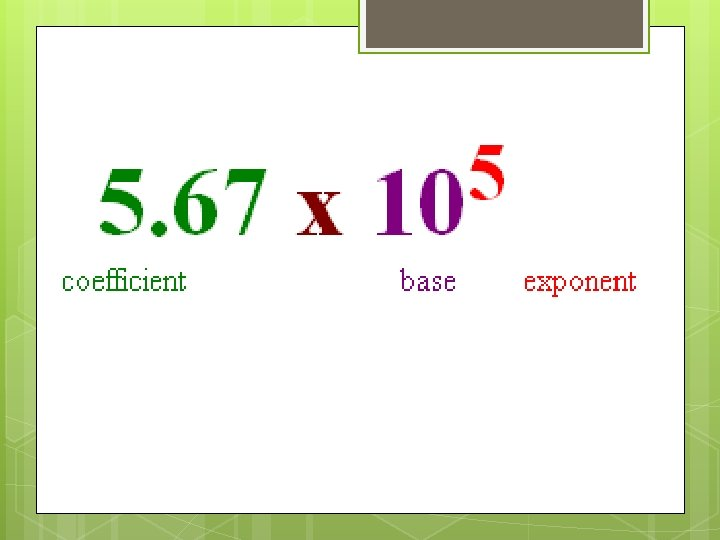Vocab:Calculations with Scientific Notation : Adding and Subtracting THINK In fractions! fractions, you must have a common denominator With scientific notation, to add or subtract, you must have a common exponent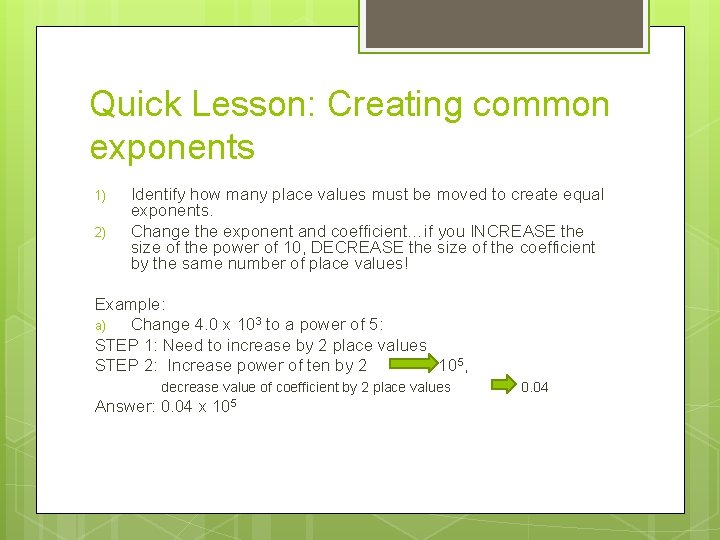Quick Lesson: Creating common exponents 1) 2) Identify how many place values must be moved to create equal exponents. Change the exponent and coefficient…if you INCREASE the size of the power of 10, DECREASE the size of the coefficient by the same number of place values! Example: a) Change 4. 0 x 103 to a power of 5: STEP 1: Need to increase by 2 place values STEP 2: Increase power of ten by 2 105, decrease value of coefficient by 2 place values Answer: 0. 04 x 105 0. 04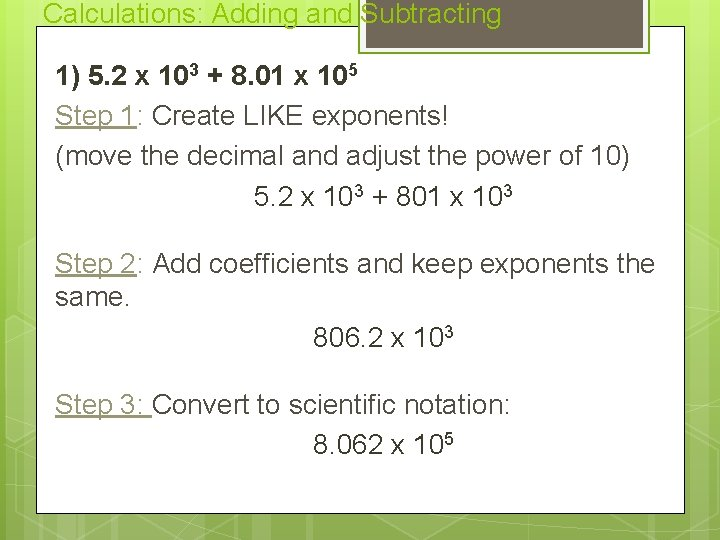Calculations: Adding and Subtracting 1) 5. 2 x 103 + 8. 01 x 105 Step 1: Create LIKE exponents! (move the decimal and adjust the power of 10) 5. 2 x 103 + 801 x 103 Step 2: Add coefficients and keep exponents the same. 806. 2 x 103 Step 3: Convert to scientific notation: 8. 062 x 105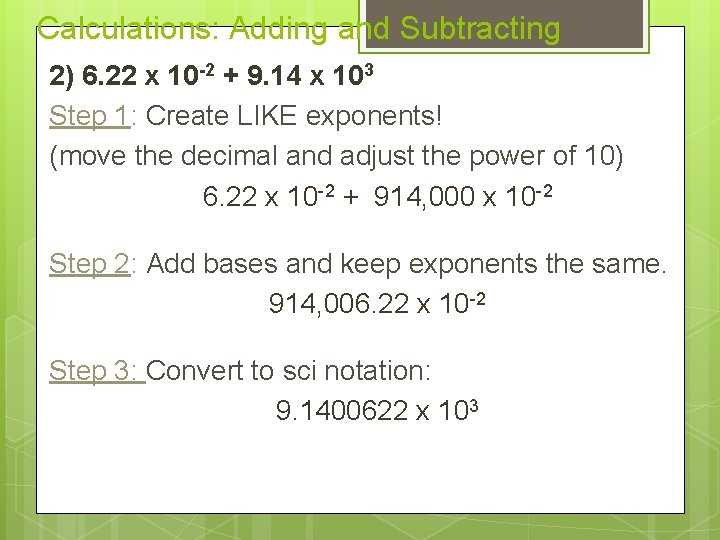Calculations: Adding and Subtracting 2) 6. 22 x 10 -2 + 9. 14 x 103 Step 1: Create LIKE exponents! (move the decimal and adjust the power of 10) 6. 22 x 10 -2 + 914, 000 x 10 -2 Step 2: Add bases and keep exponents the same. 914, 006. 22 x 10 -2 Step 3: Convert to sci notation: 9. 1400622 x 103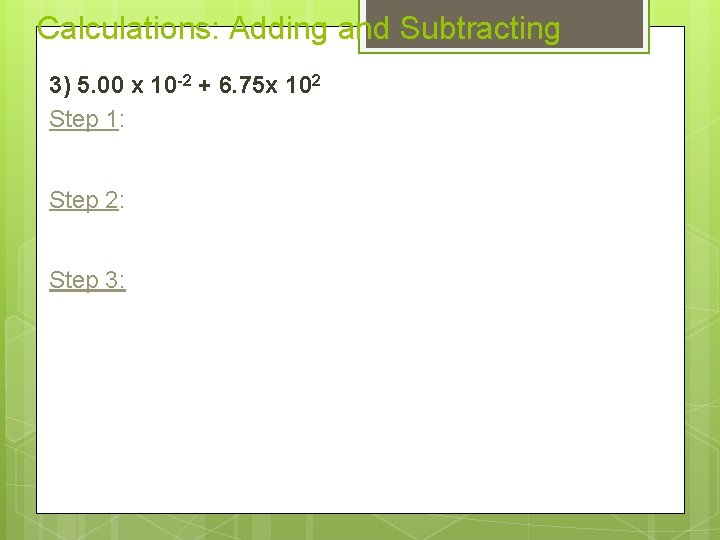Calculations: Adding and Subtracting 3) 5. 00 x 10 -2 + 6. 75 x 102 Step 1: Step 2: Step 3: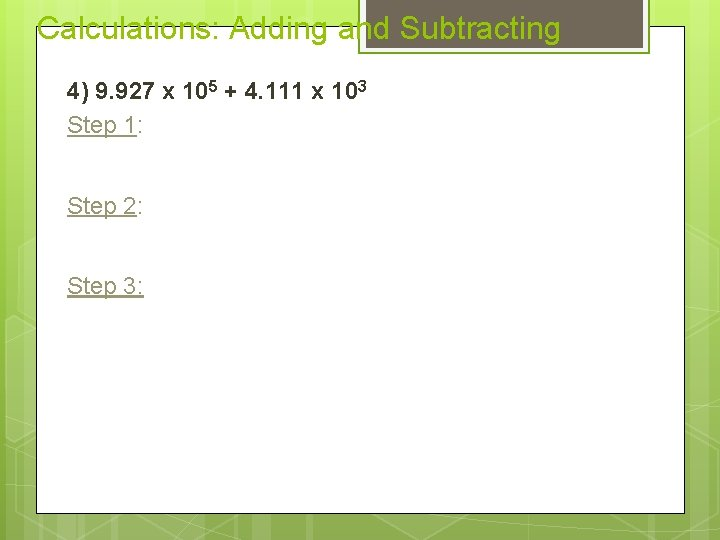Calculations: Adding and Subtracting 4) 9. 927 x 105 + 4. 111 x 103 Step 1: Step 2: Step 3: